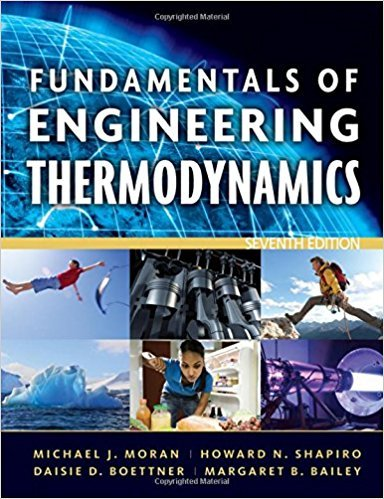×
×

# Evaluating WorkA closed system consisting of 14.5 lb ofISBN: 9780470495902 50

## Solution for problem 30P Chapter 2

Fundamentals of Engineering Thermodynamics | 7th Edition

• Textbook Solutions
• 2901 Step-by-step solutions solved by professors and subject experts
• Get 24/7 help from StudySoup virtual teaching assistantsFundamentals of Engineering Thermodynamics | 7th Edition

4 5 0 265 Reviews
28
2
Problem 30P

Evaluating Work

A closed system consisting of 14.5 lb of air undergoes a polytropic process from p1 = 80 lbf/in.2, v1 = 4 ft3/lb to a final state where p2 = 20 lbf/in.2, v2 = 11 ft3/lb. Determine the amount of energy transfer by work, in Btu, for the process.

Step-by-Step Solution:

Step 1 of 3</p>

In this problem, we have to determine the amount of energy transferred by work. The given areof air. Air undergoes a polytropic process between two specified states. The polytropic process.Step 2 of 3</p>

Since the system undergoes a polytropic process. From the pressure-volume relation for a polytropic process is.Now solve forStep 3 of 3

##### ISBN: 9780470495902

Unlock Textbook Solution